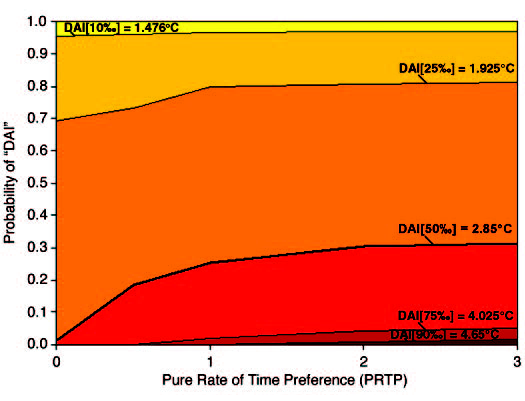Figure — The modeled relationship between the PRTP — a factor determining the discount rate — and the probability of DAI in 2100. Increasing the PRTP (and therefore the discount rate) reduces the present value of future climate damages and increases the probability of DAI[X‰] as indicated, where X is the percentile from the DAI CDF derivable from the adaptation of the IPCC Reasons for Concern figure. The solid lines indicate the percentage of outcomes above the stated threshold for DAI[X‰] for any given level of PRTP or DAI percentile threshold X. At our median threshold DAI[50‰] (thicker black line), the probability of DAI[50‰] rises from near zero with a 0% PRTP to 30% with a 3% PRTP, as originally specified in the DICE model. (Source: Mastrandrea and Schneider, 2004.)